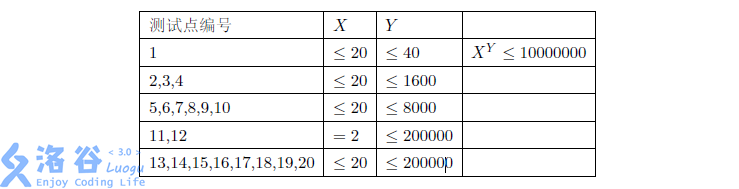# P3779 [SDOI2017]龙与地下城

• 115通过
• 574提交
• 题目提供者 deluxurous
• 评测方式 云端评测
• 标签 期望 极限 积分 各省省选 2017 山东 O2优化 Special Judge
• 难度 NOI/NOI+/CTSC
• 时空限制 1000ms-4000ms / 512MB
• 提示：收藏到任务计划后，可在首页查看。

## 题目描述

小Q同学是一个热爱学习的人，但是他最近沉迷于各种游戏，龙与地下城就是其中之一。

在这个游戏中，很多场合需要通过掷骰子来产生随机数，并由此决定角色未来的命运，因此骰子堪称该游戏的标志性道具。

骰子也分为许多种类，比如4面骰、6面骰、8面骰、12面骰、20面骰，其中20面骰用到的机会非常多。当然，现在科技发达，可以用一个随机数生成器来取代真实的骰子，所以这里认为骰子就是一个随机数生成器。

在战斗中，骰子主要用来决定角色的攻击是否命中，以及命中后造成的伤害值。举个例子，假设现在已经确定能够命中敌人，那么$YdX$（也就是掷出$Y$个$X$面骰子之后所有骰子显示的数字之和）就是对敌人的基础伤害。在敌人没有防御的情况下，这个基础伤害就是真实伤害。

众所周知，骰子显示每个数的概率应该是相等的，也就是说，对于一个$X$面骰子，显示$0, 1, 2,\dots ,X−1$中每一个数字的概率都是$\frac {1}{x}$ 。

更形式地说，这个骰子显示的数$W$满足离散的均匀分布，其分布列为除此之外还有一些性质

• $W$的一阶原点矩(期望)为$v_1(W)=E(W)=\sum_{i=0}^{X-1}iP(W=i)=\frac {X-1}{2}$

• $W$的二阶中心矩(方差)为$\mu_2(W)=E((W-E(W))^2)=\sum_{i=0}^{X-1}(i-E(W))^2P(W=i)=\frac {X^2-1}{12}$

言归正传，现在小Q同学面对着一个生命值为A的没有防御的敌人，能够发动一次必中的$YdX$攻击，显然只有造成的伤害不少于敌人的生命值才能打倒敌人。但是另一方面，小Q同学作为强迫症患者，不希望出现overkill，也就是造成的伤害大于$B$的情况，因此只有在打倒敌人并且不发生overkill的情况下小Q同学才会认为取得了属于他的胜利。

因为小Q同学非常谨慎，他会进行10次模拟战，每次给出敌人的生命值$A$以及overkill的标准$B$，他想知道此时取得属于他的胜利的概率是多少，你能帮帮他吗？

## 输入输出格式

输入格式：

第一行是一个正整数$T$，表示测试数据的组数，

对于每组测试数据：

第一行是两个整数$X$ , $Y$，分别表示骰子的面数以及骰子的个数；

接下来10行，每行包含两个整数$A$ , $B$，分别表示敌人的生命值$A$以及overkill的标准$B$。

输出格式：

对于每组测试数据，输出10行，对每个询问输出一个实数，要求绝对误差不超过$0.013579$，

也就是说，记输出为$a$，答案为$b$，若满足$|a-b|\leq 0.013579$，则认为输出是正确的。

## 输入输出样例

输入样例#1： 复制
1
2 19
0 0
0 1
0 2
0 3
0 4
0 5
0 6
0 7
0 8
0 9
输出样例#1： 复制
0.000002
0.000038
0.000364
0.002213
0.009605
0.031784
0.083534
0.179642
0.323803
0.500000

## 说明

对于$100\%$的数据，$T \leq 10$，$2 \leq X \leq 20$，$1 \leq Y \leq 200000$，$0 \leq A \leq B \leq (X-1)Y$，保证满足$Y > 800$的数据不超过$2$组。提示
标程仅供做题后或实在无思路时参考。
请自觉、自律地使用该功能并请对自己的学习负责。
如果发现恶意抄袭标程，将按照I类违反进行处理。